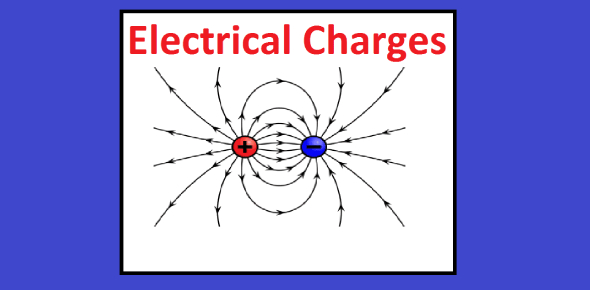# Questions On Electric Charge! Trivia Quiz

9 Questions | Attempts: 533Settings.

• 1.
A charge q is placed at the center of the line joining two equal charges Q. The system of the three charges will be in equilibrium if q is equal to
• A.

-Q/2

• B.

-Q/4

• C.

+Q/4

• D.

+Q/2

• 2.
Charge Q is divided into two parts which are then kept some distance apart. The force b/w them will be maximum if the two parts are
• A.

Q/2 each

• B.

Q/4 & 3Q/4

• C.

Q/3 & 2Q/3

• D.

None of these

• 3.
Two identical metal balls with charges +2Q & -Q are separated by some distance, & exerts a force F on each other. They are joined by a conducting wire, which is then removed. The force between them will now be
• A.

F

• B.

F/2

• C.

F/4

• D.

F/8

• 4.
Two charges, each equal to Q, are kept at x = −a, and x = an on the x-axis. A particle of mass m and charge q=-Q/2 is placed at the origin. If charge q is given a small displacement along the y-axis, the net force acting on the particle is proportional to:
• A.

-y

• B.

1/y

• C.

-1/y

• D.

Y

• 5.
Four charges, each equal to -Q are placed at the four corners of a square & a charge q is at its center. If the system is in equilibrium, the value of q is
• A.

-Q/4(1+2 √ 2)

• B.

Q/4(1+2 √ 2)

• C.

-Q/2(1+2 √ 2)

• D.

Q/2(1+2 √ 2)

• 6.
A soap bubble is given a negative charge, then its radius
• A.

Increase

• B.

Decrease

• C.

Remain unchanged

• D.

Fluctuate

• 7.
Two equal charges are separated by a distance d. A third charge placed on a perpendicular bisector at x distance from the centre will experience maximum coulomb force,when
• A.

x = (d/√2)

• B.

X = (d/2)

• C.

x = (d/2√2)

• D.

X = (d/2√3)

• 8.
Two small spherical balls each carrying a charge Q =  10 micro-coulomb are suspended by two insulating threads of equal lengths 1 each, from a point fixed in the ceiling. It is found that is equilibrium threads are separated by an angle 60º between them, as shown in the fig. What is the tension in the threads
• A.

18 N

• B.

1.8 N

• C.

0.18 N

• D.

None of the above

• 9.
Two spherical conductors B and C having equal radii and carrying equal charges on them repel each other with a force F when kept apart at some distance. A third spherical conductor having the same radius as that of B but uncharged is brought in contact with B, then brought in contact with C and finally removed away from both. The new force of repulsion between B and C is -
• A.

3F/8

• B.

3F/4

• C.

F/4

• D.

F/8

## Related TopicsBack to top
×

Wait!
Here's an interesting quiz for you.